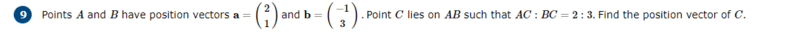• homeworkhelpls

#### homeworkhelpls

Homework Statement
Find C position vector
Relevant Equations
nonehere i found AB to be (-3, 2) and then i thought to do 2/5 multiplied by AB to find AC, however this is incorrect and instead i would have to involve the origin. Why and how can i involve the origin?

Last edited:
The problem statement in combination with your attempt are confusing for me. Can you upload a drawing of this line please? Thanks.

•vela and topsquark
here i found AB to be (-3, 2) and then i thought to do 5/2 multiplied by AB to find AC
I think AB here is supposed to be the length of the line segment connecting points A and B. It's not the vector ##\mathbf{b}-\mathbf{a}##, though it is related. And I think you meant you multiplied by 2/5, not 5/2. Or at least that would somewhat make sense.

•topsquark and berkeman
Do you know how to calculate a weighted average of vectors ##a## and ##b## so that ##a## gets 3/5 of the weight and ##b## gets 2/5? Would that point be on the AB line? How do the lengths of the AC and CB relate to the weights of the average?

Last edited:
•topsquark
Consider $\mathbf{x}(t) = (1-t)\mathbf{a} + t\mathbf{b}$ for $0 \leq t \leq 1$

•FactChecker